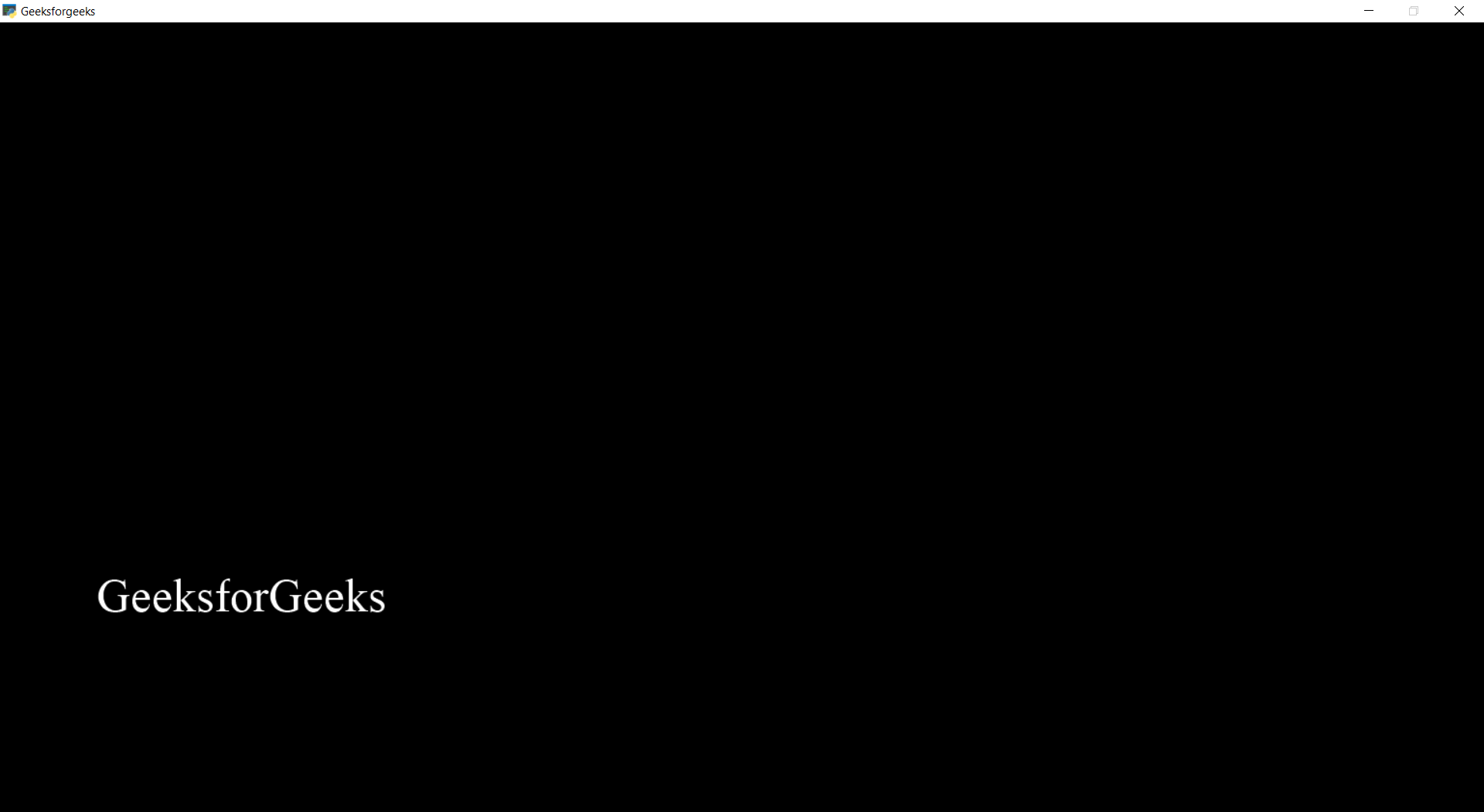# PYGLET – Maximize the Window

• Last Updated : 28 Jan, 2022

In this article we will see how we can maximize the window in PYGLET module in python. Pyglet is easy to use but powerful library for developing visually rich GUI applications like games, multimedia etc. A window is a “heavyweight” object occupying operating system resources. Windows may appear as floating regions or can be set to fill an entire screen (fullscreen). We can change the size of the window with the help of manual input although sometime there is a need to maximize the window programmatically.
We can create a window with the help of command given below

` pyglet.window.Window(width, height, title)`

In order to create window we use maximize method with the window object
Syntax : window.maximize()
Argument : It takes no argument
Return : It returns None

The behaviour of this method is somewhat dependent on the user’s display setup. On a multi-monitor system, the window may maximize to either a single screen or the entire virtual desktop.
Below is the implementation

## Python3

 `# importing pyglet module``import` `pyglet``import` `pyglet.window.key` `# width of window``width ``=` `500` `# height of window``height ``=` `500` `# caption i.e title of the window``title ``=` `"Geeksforgeeks"` `# creating a window``window ``=` `pyglet.window.Window(width, height, title)` `# text``text ``=` `"GeeksforGeeks"` `# creating a label with font = times roman``# font size = 36``# aligning it to the center``label ``=` `pyglet.text.Label(text,``                          ``font_name ``=``'Times New Roman'``,``                          ``font_size ``=` `36``,``                          ``x ``=` `window.width``/``/``2``, y ``=` `window.height``/``/``2``,``                          ``anchor_x ``=``'center'``, anchor_y ``=``'center'``)` `# on draw event``@window``.event``def` `on_draw():``    ` `    ``# clearing the window``    ``window.clear()``    ` `    ``# drawing the label on the window``    ``label.draw()``    ` `# key press event   ``@window``.event``def` `on_key_press(symbol, modifier):``    ` `    ``# key "A" get press``    ``if` `symbol ``=``=` `pyglet.window.key.A:``        ` `        ``# maximize the window``        ``window.maximize()`  `        ` `# start running the application``pyglet.app.run()`

Output :When key “A” get pressed window get maximizedMy Personal Notes arrow_drop_up